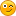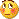# RSI indicator - page 871881

mrtools:
Whisperer, not sure if it's the best, but one of my favorites it this one here: https://www.mql5.com/en/forum/general

It really look OK

Thanks3231

whisperer:
It really look OK ThanksIt is good. I use it and it is by far the best rsi I have seen so far346

mrtools:
Drunkpips, just guessing you meant crossing 50, that's how i made this one.

pls can we have a MTF version for this indicator?183056

drunkpips:
pls can we have a MTF version for this indicator?

Multi time frame version posted here : https://www.mql5.com/en/forum/173574/page612

Files:1202

So, it seems, that sometimes it using an additional step does not necessarily mean a lag

lol, sometimes it must be magic or forex-time distortion. Can you please make a dynamic zone version of the DSS of RSI, and maybe choice of RSX, wilder's and cuttler's rsi (like DZ_PA_ IFT of RSI alert) too? Must not be mtf for me, just nmcThat would very nice and awesome. BTW, who is cuttler?183056

krelian99:
lol, sometimes it must be magic or forex-time distortion. Can you please make a dynamic zone version of the DSS of RSI, and maybe choice of RSX, wilder's and cuttler's rsi (like DZ_PA_ IFT of RSI alert) too? Must not be mtf for me, just nmcThat would very nice and awesome. BTW, who is cuttler?

I can not find who Cutler exactly isSome more information on Cutler's RSI you can find here : Relative strength index - Wikipedia, the free encyclopedia7

I have scrolled through a lot of indicators recently looking for a PLAIN WILDERS RSI - and its almost impossible to find.

I want a very ordinary RSI indi - but with one difference - its calculated as per WILDERS original specs. Not the MT4 standard one as it seems to be wrong? (as per mladen and others who noticed how Metaquotes have programmed this).

Does anyone know where the Wilders RSI is for MT4?

According to sites this i the original formula:

Wider’s RSI

The relative strength index indicator was first developed by J. Welles Wilder using an equal-weighted moving average, which was later changed to exponential. Its calculation is simple and involves constructing two series of first differences, Gain and Loss, over a period n as follows:

r(n)= close(n) – close(n-1)

If r(n) > 0 then Gain(n) = r(n), otherwise Gain(n) = 0;

If r(n) < 0 then Loss(n) = -r(n) , otherwise Loss(n) = 0;

Then, the exponential averages of the two series are taken and their ratio RS is calculated as follows:

RS = EMA(Gain(n), n)/EMA(Loss(n), n)

Finally, Wilder’s RSI is obtained after normalizing the values between 0 and 100:

RSI = 100 – 100/(1+RS)

It would be great to find the real Wilders RSI indi that should have been the standard in MT4.

Many thanks to mladen and others for the work in the thread.

Cheers183056

honda360cafe:

I have scrolled through a lot of indicators recently looking for a PLAIN WILDERS RSI - and its almost impossible to find.

I want a very ordinary RSI indi - but with one difference - its calculated as per WILDERS original specs. Not the MT4 standard one as it seems to be wrong? (as per mladen and others who noticed how Metaquotes have programmed this).

Does anyone know where the Wilders RSI is for MT4?

According to sites this i the original formula:

Wider’s RSI

The relative strength index indicator was first developed by J. Welles Wilder using an equal-weighted moving average, which was later changed to exponential. Its calculation is simple and involves constructing two series of first differences, Gain and Loss, over a period n as follows:

r(n)= close(n) – close(n-1)

If r(n) > 0 then Gain(n) = r(n), otherwise Gain(n) = 0;

If r(n) < 0 then Loss(n) = -r(n) , otherwise Loss(n) = 0;

Then, the exponential averages of the two series are taken and their ratio RS is calculated as follows:

RS = EMA(Gain(n), n)/EMA(Loss(n), n)

Finally, Wilder’s RSI is obtained after normalizing the values between 0 and 100:

RSI = 100 – 100/(1+RS)

It would be great to find the real Wilders RSI indi that should have been the standard in MT4.

Many thanks to mladen and others for the work in the thread.

Cheers

Check this post : https://www.mql5.com/en/forum/173574/page459

That is the original Welles Wilder RSI calculation7

Check this post : https://www.mql5.com/en/forum/173574/page459 That is the original Welles Wilder RSI calculation

Thanks mladen. Just confirming it is the TrendRSI 2 indi listed at that link you provided?7

I did also find a RSI_Multi indi at this link on this forum:

It has 4 inputs as to moving average used in RSI calc - 0 SMA, 1 EMA, 2 Wilders MA, 3 Weighted MA.

Was hoping that RSI code was correct.

Cheers